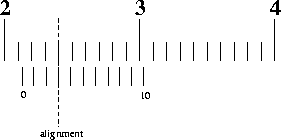# 150MM VERNIER CALIPER WITH WOODER BOX

GAMMA

SG - VC0309

30

S\$ 12.00

## Instructions on use

• The Vernier caliper is an extremely precise measuring instrument; the reading error is 1/20 mm = 0.05 mm.
• Close the jaws lightly on the object to be measured.
• If you are measuring something with a round cross section, make sure that the axis of the object is perpendicular to the caliper. This is necessary to ensure that you are measuring the full diameter and not merely a chord.
• Ignore the top scale, which is calibrated in inches.
• Use the bottom scale, which is in metric units.
• Notice that there is a fixed scale and a sliding scale.
• The boldface numbers on the fixed scale are centimeters.
• The tick marks on the fixed scale between the boldface numbers are millimeters.
• There are ten tick marks on the sliding scale. The left-most tick mark on the sliding scale will let you read from the fixed scale the number of whole millimeters that the jaws are opened.• In the example above, the leftmost tick mark on the sliding scale is between 21 mm and 22 mm, so the number of whole millimeters is 21.
• Next we find the tenths of millimeters. Notice that the ten tick marks on the sliding scale are the same width as nine ticks marks on the fixed scale. This means that at most one of the tick marks on the sliding scale will align with a tick mark on the fixed scale; the others will miss.
• The number of the aligned tick mark on the sliding scale tells you the number of tenths of millimeters. In the example above, the 3rd tick mark on the sliding scale is in coincidence with the one above it, so the caliper reading is (21.30 ± 0.05) mm.
• If two adjacent tick marks on the sliding scale look equally aligned with their counterparts on the fixed scale, then the reading is half way between the two marks. In the example above, if the 3rd and 4th tick marks on the sliding scale looked to be equally aligned, then the reading would be (21.35 ± 0.05) mm.
• On those rare occasions when the reading just happens to be a "nice" number like 2 cm, don't forget to include the zero decimal places showing the precision of the measurement and the reading error. So not 2 cm, but rather (2.000 ± 0.005) cm or (20.00 ± 0.05) mm.

## GET THE LATEST NEWS DELIVERED DAILY

Give us your email and you will be daily updated with the latest events, in detail.